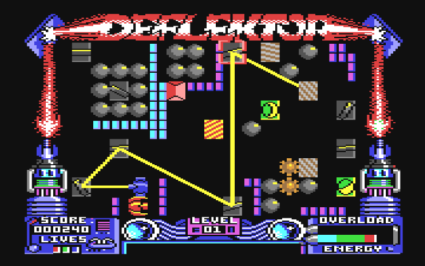# Deflektor

A single-player game where the player orients mirrors to direct a laser to a target.## Description

Deflektor is a single-player puzzle game. A level is a grid of static elements, one of them emits a laser beam whose trajectory is affected by the other elements it encounters. The goal is to “collect” a set of items by making the beam hit each one, not necessarily at the same time. Items can be collected in any order.

The player can only control the orientation of a set of mirrors. When the beam encounters one of these mirrors, it is reflected so that the angle of incidence equals the angle of reflection. Each mirror has 16 possible orientations.

Other static elements (which cannot be interacted with by the player) are opaque tiles that absorb the beam, and teleporters that absorb the beam and emit it again from another location in the level. Some tiles rotate automatically at regular intervals. They are self-rotating mirrors, and polarization filters, which let the light cross them in only one direction. Self-rotating objects simultaneously rotate through their 8 possible orientations at regular intervals.

Finally, there is a random element in the gameplay: elements called prisms will redirect the beam changing its direction regularly and randomly, and enemies (gremlins) which randomly appear, attach to mirrors, and change their orientation.

## Computational complexity

 proves that the problem of deciding whether a Deflektor level is solvable is in L (i.e., it can be solved in logarithmic space w.r.t. the number of elements in the level and the number of items to collect), via a reduction from the undirected connectivity problem.

The sketch of the proof is the following:

1. Build a reachability graph $G_i$ for each of the 8 orientations of the (self-)rotating game elements, such that two objects are connected in $G_i$ if one can redirect a beam to the other. Among others, each of these graphs has a vertex $r$ representing the beam emitter.

2. Build a graph $G’$ consisting of the disjoint union of all the $G_i$s, except for a single common beam emitter vertex $r$.

3. Create $k$ copies of $G’_1, \dots, G’_k$ of $G’$, where $k$ is the number of items to collect.

4. For all $j=1, \dots, k-1$, link all vertices representing the $j$-th item to collect in $G’_j$ to the beam vertex of $G’_{j+1}$.

5. Connect all vertices representing object $k$ in $G’_k$ to a single exit vertex $t$.

The level is solvable (i.e, all collectible items can be reached by the beam) if and only if therere exists a path from the beam emitter $r$ of $G’_1$ to $t$ in the resulting graph.

 Giovanni Viglietta. “Gaming is a hard job, but someone has to do it!” In: Theory of Computing Systems 54.4, 2014.

@misc{cog:deflektor,
author = "{CoG contributors}",
title  = "{Deflektor --- Complexity of Games}",
year   = "2022",
url    = "https://www.isnphard.com/i/deflektor/",
note   = "[Online; accessed 2022-06-02]"
}